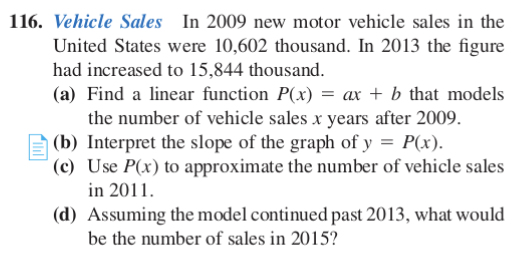# 116. Vehicle Sales In 2009 new motor vehicle sales in theUnited States were 10,602 thousand. In 2013 the figurehad increased to 15,844 thousand.(a) Find a linear function P(x) = ax + b that modelsthe number of vehicle sales x years after 2009.A (b) Interpret the slope of the graph of y = P(x).(c) Use P(x) to approximate the number of vehicle salesin 2011.(d) Assuming the model continued past 2013, what wouldbe the number of sales in 2015?

Question
8 views

116c) and 116d)help_outlineImage Transcriptionclose116. Vehicle Sales In 2009 new motor vehicle sales in the United States were 10,602 thousand. In 2013 the figure had increased to 15,844 thousand. (a) Find a linear function P(x) = ax + b that models the number of vehicle sales x years after 2009. A (b) Interpret the slope of the graph of y = P(x). (c) Use P(x) to approximate the number of vehicle sales in 2011. (d) Assuming the model continued past 2013, what would be the number of sales in 2015? fullscreen
check_circle

Step 1

Part (a):

Step 2

Substitute a and point in linear equation.

Step 3

Part (c):

...

### Want to see the full answer?

See Solution

#### Want to see this answer and more?

Solutions are written by subject experts who are available 24/7. Questions are typically answered within 1 hour.*

See Solution
*Response times may vary by subject and question.
Tagged in

### Other